# 民用高层建筑翻弯跨越交叉管道应力研究Research on Stress of Turning over Crossing Pipes in Civil High-Rise Buildings

• 全文下载: PDF(1154KB)    PP.27-35   DOI: 10.12677/HJCE.2019.81005
• 下载量: 398  浏览量: 532

The complicated pipelines of civil high-rise buildings can easily lead to the problem of crossing different pipelines in the horizontal plane. And turning over the intersecting pipeline becomes a more reasonable countermeasure. Therefore, it is quite necessary to carry out research on the pipeline stress distribution of the above method. In this paper, the stress distribution of pipelines spanning 90˚, 120˚ and 135˚ is studied. It is concluded that the maximum stress point is the front and rear straight pipe supporting hanger points, and when the turning angle is increased, the stress level is gradually reduced, and the 135˚ turning is the best choice for the purpose of limiting the primary stress. Under the same temperature condition, the larger the turning angle is, the larger the secondary stress is, so as to limit the secondary stress. 90˚ turning is the best choice for the purpose.

1. 引言

2. 工况参数确定与模型建立

2.1. 管道基本参数确定

2.2. 载荷工况确定Table 1. Basic parameters of the pipelineTable 2. Combination of Pipe load cases

2.3. 物理模型建立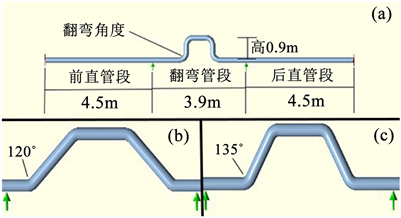Figure 1. (a) 90˚ upturned pipe section and overall model of the pipeline; (b) 120˚ upturned pipe section model diagram; (c) 135˚ upturned pipe section model diagram

2.4. 管应力计算模型

${S}_{L}={\left[{I}_{a}{\left(|{S}_{lp}+{F}_{ax}/{A}_{p}|+{S}_{b}\right)}^{2}+4{S}_{{}_{t}}^{2}\right]}^{1/2}<{S}_{h}$ (1)

${S}_{E}={\left[{\left(|{i}_{a}{F}_{ax}/{A}_{p}|+{S}_{b}\right)}^{2}+4{S}_{{}_{t}}^{2}\right]}^{1/2} (2)

SL为综合应力；

Slp为纵向压应力，计算公式为：

${S}_{lp}=P{D}_{i}^{2}/\left({D}_{o}^{2}-{D}_{i}^{2}\right)$ (3)

Di、Do分别为管道内直径与外直径；

Fax为轴向力；

Ap为管道横截面积；

Ia为持续轴向应力系数；

Sh为材料在设计温度下许用应力；

Sc为环境温度下许用应力；

Sb为弯曲应力，计算公式为：

${S}_{b}={\left[{\left({I}_{i}{M}_{i}\right)}^{2}+{\left({I}_{o}{M}_{o}\right)}^{2}\right]}^{1/2}/Z$ (4)

Mi、Mo分别为平面内、外弯矩；

Ii、Io分别为平面内、外应力增强系数；

Z为管截面模量；

St为扭转应力，计算公式为：

${S}_{t}={I}_{t}{M}_{t}/2Z$ (5)

Mt为扭转力矩；

It为扭转力矩系数。

3. 计算结果分析

3.1. 管道翻弯应力总体分析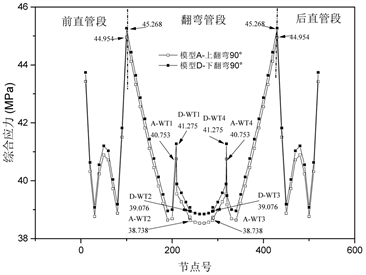Figure 2. Comprehensive stress distribution diagram of 90˚ up and down turning under L1 load conditions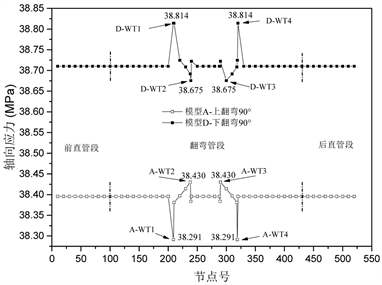Figure 3. Axial stress distribution diagram of 90˚ up and down turning under L1 load conditions

3.2. 翻弯角度影响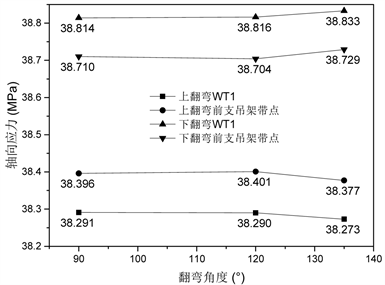Figure 4. Variation diagram of axial stress varied with turning angle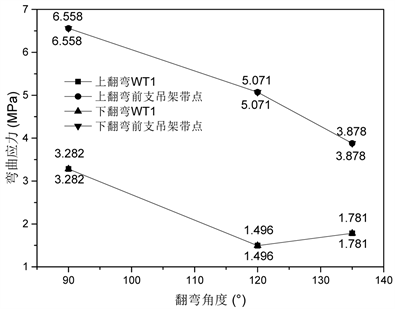Figure 5. Variation diagram of bending stress varied with turning angle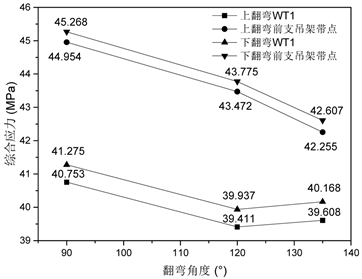Figure 6. Variation diagram of comprehensive stress varied with turning angle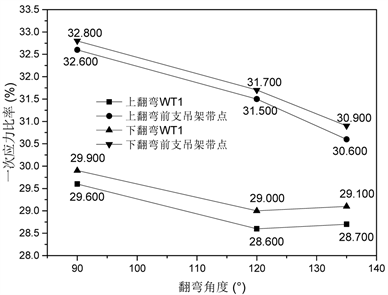Figure 7. Variation diagram of primary stress ratio varied with turning angle

3.3. 温度的影响Figure 8. Variation diagram of secondary stress ratio varied with temperature in the support hanger point of the front straight pipe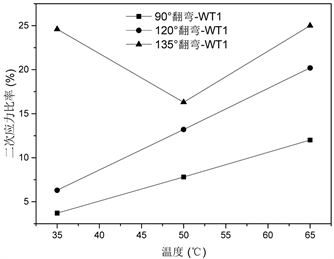Figure 9. Variation diagram of secondary stress ratio varied with temperature in the support hanger point of the WT1

4. 结论

1) 由应力水平可以看出，一次应力薄弱点为前后直管段支吊架点，二次应力薄弱点为弯头WT1与WT4。对于已经建成的管道翻弯而言需要进行重点关注。

2) 随着翻弯角度的增大，前后直管段支吊架点代表的一次应力最大值上呈现下降趋势而弯头WT1与WT4点则在120˚取得最小值，因此选择大翻弯角度有利于降低一次应力水平。

3) 同等温度条件下，翻弯角度越大，二次应力越大。因此选择小翻弯角度有利于降低二次应力水平。

4) 总体上随着温度的上升，二次应力呈现增大的趋势。但135˚翻弯在小于50℃下呈现相反变化趋势，值得进一步研究。

  王成洋, 白蓉, 蒋韶鑫, 孙方荣. 超高层核心筒墙帽与空心钢管劲性复合楼盖施工技术[J]. 土木工程, 2013, 2(1): 43-48.  张延斌. 浅谈市政排水管道施工中管线交叉处理措施[J]. 科技资讯, 2010(25): 91-91.  张伟中. 市政管道交叉冲突的处理方法[J]. 建材与装饰: 上旬, 2018(27): 23-24.  张雨飞, 卢志旋. 市政管道交叉冲突的处理方法[J]. 给水排水, 2003, 29(10): 87-89.  刘丰, 于银海. 国内外海底管道交叉点处理方法研究[J]. 中国石油和化工标准与质量, 2018(7): 120-121.  霍文星. 斜拉跨越技术在海底管道交叉铺设中的应用[C]//中国造船工程学会近海工程学术委员会, 中国海洋学会海洋工程分会, 中国石油学会海洋石油分会, 中国高科技产业化研究会海洋分会, 台湾中国土木水利工程学会海洋工程委员会. 2014年第三届中国海洋工程技术年会论文集: 2014年卷. 中国造船工程学会近海工程学术委员会, 中国海洋学会海洋工程分会, 中国石油学会海洋石油分会, 中国高科技产业化研究会海洋分会, 台湾中国土木水利工程学会海洋工程委员会, 2014: 5.  雷新义. 直埋供热管道上翻跨越相交管线的研究[J]. 区域供热, 2013(4): 105-108.  工艺管道[S]. US-ANSI, 2016.